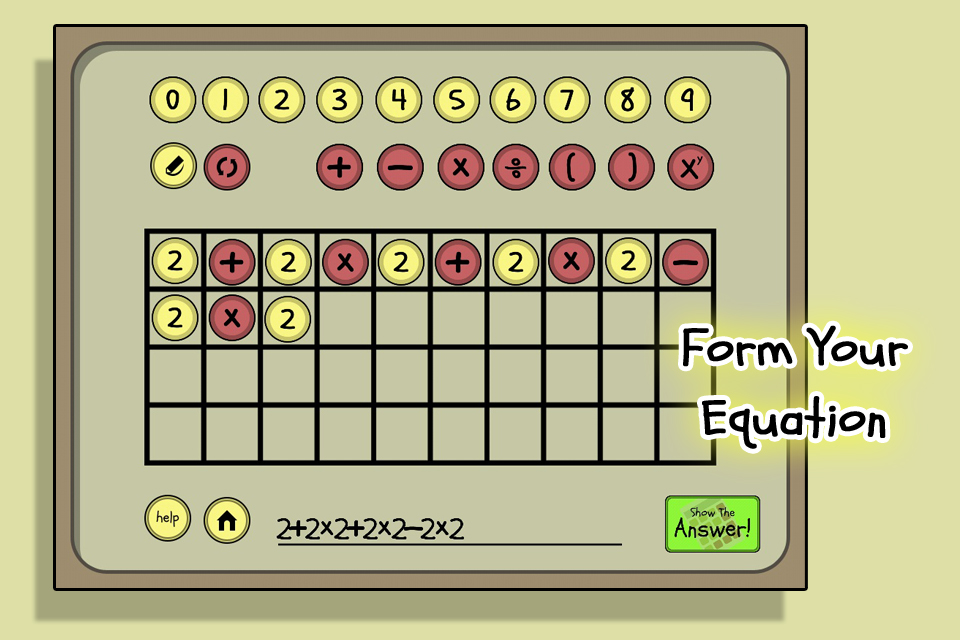# OoO Calc: Order of Operations Calculator (App)Order of Operations Calculator is a calculator that shows you the solution step by step so you can learn while using a calculator.

### Operations and Algebraic Thinking

CCSS Math: 5.OA.A.1 – Use parentheses, brackets, or braces in numerical expressions, and evaluate expressions with these symbols.## 2 + 2 x 2 + 2 x 2 – 2 x 2 =

a. 6

b. 16

c. 40I was absolutely astonished that so many people had got the answer wrong. You can find the answer below if you are one of the many who need a little brushing up on how the order of operations work.The calculator follows the standard order of operations taught by most algebra books – Parentheses, Exponents, Multiplication and Division, Addition and Subtraction. When using this calculator you will see a step-by-step solution instead of just the answer.#### STEPS FOR ORDER OF OPERATIONS

Step 1 : Do operations in parentheses or (brackets) and other grouping symbols first.

Step 2 : Simplify orders(powers and square Roots), working from left to right.

Step 3 : Do multiplication and division operations from left to right.

Step 4 : Do addition and subtraction operations from left to right